Metabolism calculators
• METABOLIC ALLOMETRY...    'm'   Use this somewhat specialized utility to make estimates of an animal's resting metabolism, based on its size and taxonomic affiliation.  The metabolism calculator has many potential uses; for example, you might want to use it as a 'reality check' if you think your own metabolic data are unexpectedly high or low.
The initial popup menu contains some very generalized equations, and also allows you to switch to submenus for specific taxa (arthropods, fish, birds, mammals, etc.).  For most taxa, several different equations are available (from different literature sources (listed here).  You can also pick the desired output units.  The energy equivalence of metabolism (joules per ml of oxygen consumed) can be set with the 'O2 heat equivalence' selection in the "Respirometry" submenu (EDIT menu); the default value is 20.1 joules/ml.  The mass coefficient in the allometric equation ('a' value) is adjusted to reflect whatever output unit is in use.  Results can be stored for later use.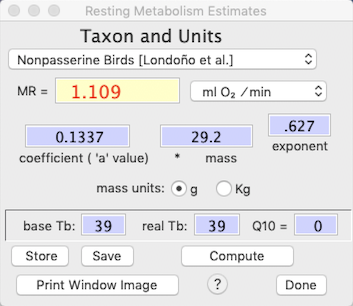Metabolism for all taxa are calculated from power functions:

metabolism = a Mb

(where a is the mass coefficient, M is mass, and b is the mass exponent)

This example shows an estimate of the resting metabolic rate (RMR) of a 37.3 g bird, in units of ml O2/min.  The equation was derived from a paper published by Andrew Mckechnie and Blair Wolf (Click here for a list of the references from which allometric equations were obtained.).
Note that the mass coefficient ('a' value) and mass exponent are shown and can be edited, and that mass can be in either grams or kilograms.  Also, it is possible to make corrections for the effect of body temperature by making the appropriate adjustments to the value of Tb and Q10 (in this example, the 'base' Tb, from which the equation was derived, is equal to the current Tb so no temperature correction occurs).  After changing values in the edit fields, click the 'Compute' button to display the new results.

The 'Store' button 'remembers' the computed metabolism for later use (for example in other calculators).   The 'Save' button, if present, lets you save the current mass coefficient and mass exponent values for future use, accessed as 'Custom coefficent and exponent' option in the Taxon popup.   The 'Save' button is accessible only if the units are set to ml O2/min.   NOTE:   you will have to click the 'Save Current Preferences' button in the Preferences window if you want to have your custom values available the next time you run the program.

•    MINIMUM FLOWRATE...      This is similar to the Metabolic allometry calculator, but it also makes an estimate of the flow rate necessary to maintain an acceptable oxygen concentration within a metabolism chamber.  This example shows a calculation for a small shark in brackish water (since the osmolarity of water affects its oxygen capacity).   Note that the osmolarity popup appears only for fish metabolism.   Also keep in mind that the calculation is based on a user-entered maximum change in gas concentration or percent saturation, not on the absolute concentration or partial pressure of oxygen.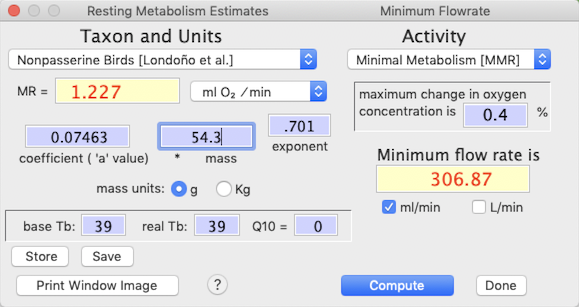You can also adjust the activity intensity for the animal, ranging from inactive (minimal metabolism; MMR) to average daily metabolism (3 X MMR) to very vigorous activity -- up to 100X MMR, which is reasonable for some large flying insects.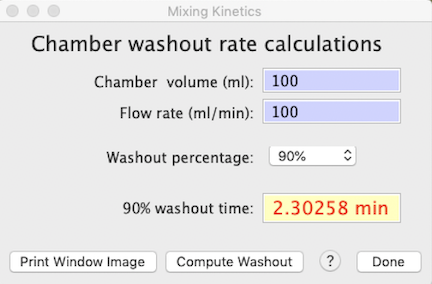•   CHAMBER WASHOUT…     All metabolic chambers act as 'mixing boxes' for respiratory gases and the incurrent flow of respiratory fluid (gas or water).  Unless flow rates are extremely high relative to chamber volume (in which case it may be difficult to attain a sufficent change in respiratory gas concentrations to measure accurately), the excurrent gas concentrations are integrated averages of the 'instantaneous' metabolic rate of the animal over time.   This is mostly irrelevant if metabolic rate is constant and you have plenty of time between baseline reference readings, but instantaneous changes (or reference readings) in metabolism produce exponential approaches to the new value, not a step change.   The washout kinetics of the chamber are crucial (along with how well a chamber is mixed) in determining the response time to changes in metabolic rates.

This window calculates the washout rates of theoretical perfectly-mixed chambers as a function of volume and flow rate.  The computed value is the time for X% of intial gas volume to be replaced -- akin to the 'half-life' concept for radioactive decay and related phenomena. back to top

•   Q10 ADJUSTMENT…     This calculates the effect of temperature (Q10) on the rate of reactions or functions -- speed, power, metabolism, etc.   To do that, enter a base temperature and an adjusted temperature, the base value of the rate function, and the Q10 (the default is 2.2), and click the 'compute adjusted value' button.   Alternately, enter the base and adjusted temperatures and the base and adjusted rate values, and compute Q10 by clicking the 'compute Q10' button.   Q10 is the factorial change in rate across a 10 °C temperature change.   The temperature difference (base temperature to adjusted temperature) can be positive or negative.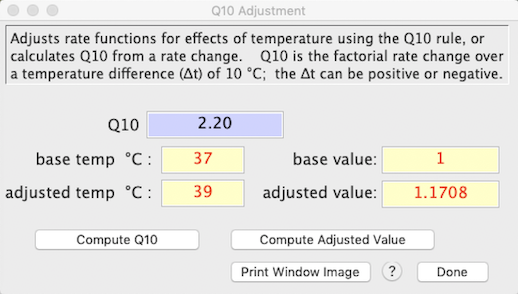•    CLOSED SYSTEM RESPIROMETRY...     This calculator computes rates of oxygen consumption (VO2) and/or carbon dioxide production (VCO2) in air or other breathable gas mixture in a closed system (i.e, the animal is sealed in an air-tight chamber for some time and metabolism is computed by the change in concentration of O2 and CO2 between initial and final gas samples).   You need to enter:
• chamber temperature, pressure, and relative humidity (when sealed, not at the end of measurements).
• chamber volume
• elapsed time (between taking the initial and final gas samples)
• initial fractional concentrations of O2 (FiO2) and CO2 (FiCO2)
• the change in concentration (in percent) of oxygen and/or CO2, and the respiratory quotient (RQ)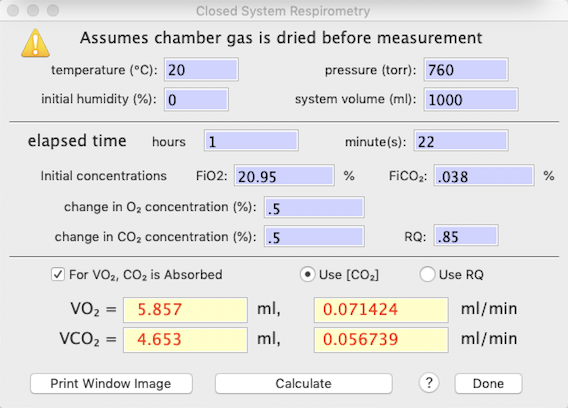If you measure only one of these two gas species, the program will use RQ to estimate the other.   If you measure both O2 and CO2 concentration changes, the program will optionally use O2 in the calculation of VCO2. NOTE: it is assumed that sample gas is dried before measurement, and the default settings are:
• Initial O2 concentration (FiO2) = .2095
• Initial CO2 concentration (FiCO2) = .0004
• CO2 is absorbed prior to O2 measurement
• VCO2 is computed from VO2, if oxygen is measured, or from RQ otherwise

Equations notes:
• STP = standard temperature and pressure (0°C, 760 torr)
• delta[O2] = fractional change in O2 concentration
• delta[CO2] = fractional change in CO2 concentration
• FeO2 = FiO2 - delta[O2]
• FeCO2 = FiCO2 + delta[CO2]
VO2 equations:
• CO2 is absorbed: VO2 = STP volume * delta[O2] /(1 - FeO2)
• CO2 NOT absorbed, compute from delta[CO2]: VO2 = STP volume * (delta[O2] - (FeO2 * delta[CO2])/(1 - FeO2)
• CO2 NOT absorbed, compute from RQ: VO2 = STP volume * (delta[O2] /(1 - FeO2 * (1-RQ))
VCO2 equations: NOTE: at typical delta[CO2], different equations have little effect on calculated VCO2
• from RQ: VCO2 = STP volume * delta[CO2]/(1 - FeCO2 * (1-(1/RQ)))
• from VO2: VCO2 = STP volume * (delta[CO2] - (FeCO2 * VO2))/(1 - FeCO2)

•    VENTILATION...    'v'    This rather arcane calculator is for studies of ventilation (breathing dynamics) using open-flow plethysmography systems.  It will compute tidal volume (Vt, the volume of gas inspired with each breath), minute volume (Vmin, the total volume of gas inspired each minute), and oxygen extraction (EO2, the fraction of inspired oxygen that is absorbed from tidal gas).

To support these calculations -- which are largely based on the small pressure fluctuations induced by the warming and wetting of tidal air -- you need to provide a number of variables.  Several of these are self-explanatory (at least if you know something about respiratory physiology).  Abbreviations for some of the more obscure ones are:

• VO2, the rate of oxygen consumption
• Tb and Ta (body and air temperature)
• delta-P (pressure gradient between the metabolism chamber and ambient barometric pressure, in mm of water)
• RH (relative humidity in the metabolism chamber)
• calibration vol (the volume of gas injected during system calibrations)
• calibration volts (mean peak height of calibration deflections)
• calibration gain (signal amplification during calibrations)
• sample volts (mean peak height of individual breaths in the breathing record)
• sample gain (signal amplification when recording breathing).

You can use the 'waveform analysis' routines in the ANALYSIS menu to obtain breathing frequeny, calibration volts, and sample volts from recorded breathing records.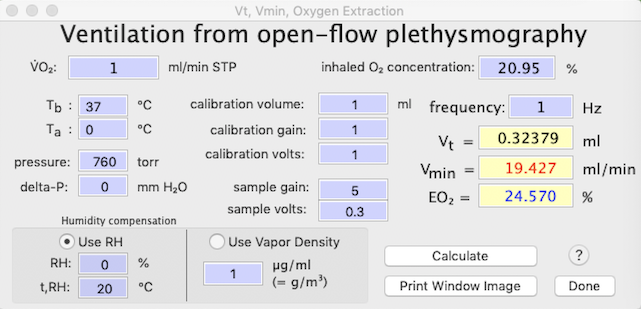In this fairly typical example, the animal (a mouse) breathed about 6.3 times per second (not unusual for a small mammal in cold conditions) and had a tidal volume of 0.256 ml and a minute volume of about 97 ml/min.  The oxygen extraction was about 25.7%.

• Note that you can use either relative humidity (RH) + measurement temperature (T,RH) or water vapor density to compute the water vapor component of the equations.

Although there are a lot of data to enter, the program makes it as easy as possible.  Most values are remembered between successive uses of the calculator, so you only have to change a few edit fields (like VO2, frequency, and sample volts).  You can tab (or hit return) to move between successive edit fields.

•    THERMOREGULATORY COSTS…      This specialized calculator, of interest mainly to thermal physiologists, uses weather (temperature) data from a loaded file to estimate the energy cost of thermoregulation for an endotherm:   e.g., bird or mammal.   The calculations produce a mean cost of thermoregulation, plus several related values, over whatever period the weather data encompass.

 Important cautions: For temperature data, the program's default 'assumption' is that a value of zero = bad data (or no data).  If your temperatures include real values of zero, then use .001 (or some other small but non-zero number) instead of zero. The program will attempt to find the highest 6 and 12-h readings, so the implicit assumption is that your climate data are sampled at least once per hour (higher sampling rates are OK).

Thermoregulatory costs are calculated using the following thermal parameters:   body temperature (Tb), lower critical temperature (LCT), thermal conductance (Cth; watts/°C), and basal metabolic rate (BMR; ml O2/min or watts; selected in the 'options' window) in a set of edit fields.   The program also asks for a channel containing time of day in hours (0-24) and two channels containing environmental temperatures (Te), one for shade and one for sun.   Note: If shade temperature is missing, the program can substitute sun temperature (if available), but only at night.   If sun temperature is missing, the program can substitute shade temperature (if available).   Select these settings in the Data Rejection Rules component of the Thermoregulatory Cost Options menu.

For each point in the data file, the necessary metabolic rate is computed according to the following rules:

• if the animal is day-active, whichever Te (sun or shade) is highest is used in calculations.
 Note that if you have only one temperature measurement, or if a day-active species never has access to sunlight (e.g., stays in deep shade), you can select the shade temperature as the sun temperature (in other words, use the shade temperature measurement twice.
• at Te >= LCT, metabolic rate is set equal to BMR (internally converted to watts)
• at Te < LCT, metabolic rate is computed as: (Tb - Te) * Cth

When Te < LCT in the inactive part of the daily cycle, if the animal can use torpor (selected in the 'options' window), one of two calculations are performed:

-- if Te is at or above 1 °C less than a minimal (defended) Tb, the metabolic rate is reduced from BMR in proportion to how close Te is to minimum Tb.
-- if Te is lower than 1°C below the minimum Tb, metabolic rate is computed as: (minimum Tb - Te) * Cth
-- NOTE:   the torpor algorithms do not include the energy cost of warmup.

Flowcharts (decision trees) for calculations of environmental temperature and thermoregulatory costs are at the end of this page.

• Program output includes:

• mean metabolic rate (watts)
• factorial increase of mean metabolic rate above BMR
• highest single metabolic rate (watts)
• factorial increase of highest metabolic rate above BMR
• percent of total samples for which metabolic rate = BMR (i.e., Te > LCT)
• mean metabolic rate for all samples where Te < LCT
• factorial increase above BMR for mean metabolic rate for all samples where Te < LCT
• maximum daily average (watts), and expressed as factorial increase above BMR
• maxima over 6 and 12 hours (watts), and expressed as factorial increase above BMR
• mean metabolic rate while in torpor (watts), and expressed as factorial change from BMR
• percent of time spent in torpor

 NOTE:   For nightime data, if a value for shade temperature is missing, the program will attempt to use the sun temperature value instead.  This substitution does not occur for daytime data.

•  These thermal calculations are performed in one of two ways: One individual at a time (click the 'compute costs' button):   The user enters the animal's thermal parameters in edit fields in the main program window, selects the time, sun, and shade temperature channels, and then starts calculations.  Results are shown after all Te data are processed: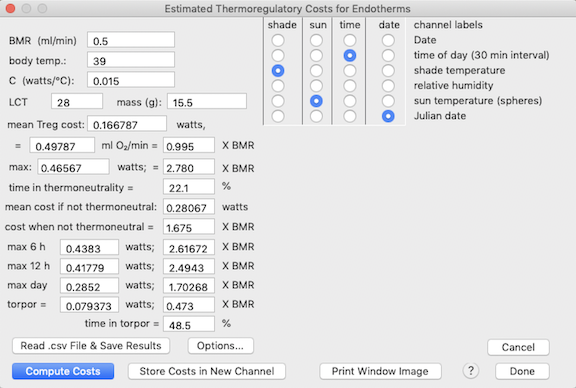Optionally, the computed costs for each entry in the main data file can be saved in a new channel (if the file has < 40 channels). Multiple individuals (species) in a spreadsheet (click the 'read .csv file and save results' button):   The user selects the time and two Te channels (sun and shade) and then opens a spreadsheet file (comma separated variables; .csv) containing a series of thermal parameters for different species, sexes, etc.   The maximum number of variables in the .csv spreadsheet is 50.   Each row of the spreadsheet (up to 800) contains data for one individual or species.  You need to select the columns that contain: an indicator (yes, no, y, n, 1, 0) of whether the animal can 'use' sunlight to warm itself.   If true, the calculations use the 'sunshine' value in the main data file of temperature data. body temperature, in degrees C minimum thermal conductance, in watts/degree C (here, degrees C is the gradient between body temperature and environmental temperature). the Lower Critical Temperature (LCT) in degrees C. Basal Metabolic Rate (BMR); the units are either watts or ml O2/min (selected in the 'options' window). an indicator (yes, no, y, n, 1, 0) of whether the animal can use torpor to save energy during the inactive phase (night or day) of its daily cycle. the minimum body temperature (Tb; degrees C) that the animal will tolerate in torpor.   The lowest environmental temperature at which this is achieved without increasing metabolism is assumed to be 1 °C lower than minimum Tb.  At lower Te, the animal will increase metabolic heat production to maintain Tb at the minimum value.   The program uses the thermal conductance value (Cth) to calculate this metabolic rate. an indicator (yes, no, y, n, 1, 0, D, d, N, n) of whether the animal's active phase is during the day (diurnal) or at night (nocturnal).   A 'yes' or '1' value or equivalent means the animal is diurnal.For each spreadsheet entry, the program runs through the Te channels from the main data file. The combined results can be saved, along with the raw data from the 'source' .csv file, in a user-selected spreadsheet file. The program always saves a column containing the mean thermoregulatory cost in watts. Other thermoregulatory data columns to be placed in the spreadsheet are selected from the window at right:
For both methods, the 'options' button opens a window where you can select a number of alternate ways of handling data and calculations, including: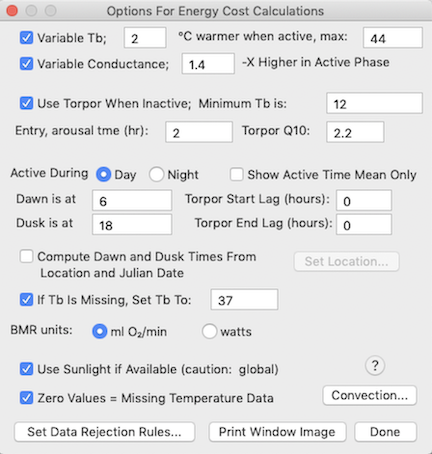• the units for basal metabolic rate (BMR):   watts or ml O2/min.

• whether to compute results for active time only (and what the hours of daylight are).   Alternately, you can get active time information from channel in a .csv data file.   Currently the hours of daylight are not obtainable from a .csv file and must be entered here.

• whether to allow for a different euthermic body temperature during the active phase versus the inactive phase (day or night); the value in the data file (or in the main 'Thermoregulatory costs' window) is assumed to be the inactive phase body temperature.

• whether thermal conductance also changes with the daily activity cycle (and if so, how much); the default value is a 1.4X higher conductance during the active phase.

• a default value of body temperature if that data is missing in a .csv file entry.

• whether to compute results assuming the animal becomes torpid during the inactive time of day (in a .csv data file this can be indicated by 'yes', 'no', or 'N' or 'n' and 'D' or 'd', or 0 and 1).

• If torpor is used, the duration of the entry and arousal phases (body temperature is 'ramped' for calculations during these periods), the minimum defended body temperature while in torpor (this can be entered as a variable in a .csv file), and the Q10 value for adjusting metabolic rate during torpor.

• If torpor is used, the time lags during the inactive phase for the onset and completion of torpor (which often does not occupy the entire inactive phase).

• If torpor is used, the duration of the entry and arousal phases (body temperature is 'ramped' for calculations during these periods), the minimum defended body temperature while in torpor (this can be entered as a variable in a .csv file), and the Q10 value for adjusting metabolic rate during torpor.

 Note that if you elect to NOT allow torpor use, that restriction will apply globally to all entries in a .csv file.   If you DO allow torpor use, each entry in a .csv file will indicate whether or not torpor is applicable to that entry.

• Whether a zero value for temperature is treated as missing data (and ignored); the alternate acceptable missing data indicator is a value of -9999.

• Whether to use constant values for sunrise and sunset times, or compute them from location and Julian date (the day of the year from 1-365; 366 in a leap yaar).   You will need to enter a location in latitude and longitude (fractional degrees, not degrees and minutes), and the data file with temperature information will need a veriable showing Julian date.Times are computed using the ‘Sunrise equation’; this example shows an annual day length cycle for a tropical latitude (~ 14 ° south).

The following flowchart shows how environmental temperature (Te) is selected.   NOTE: the 'sphere' temperature is the temperature of a life-sized model of the animal (often a painted metal or plastic sphere) placed in the habitat where it is affected by wind and sunlight -- in the absence of sunlight or some other strong radiant source or sink, sphere temperature ( Tsphere) is the same as air temperature:This flowchart shows how Te, time, and physiological parameters are used to compute thermoregulatory energy costs: